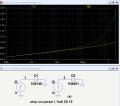# Is it possible to calculate in a circuit, the Ohmic resistance of a diode

#### ouzcoskun

Joined May 12, 2016
32
Is it possible to calculate in a circuit, the Ohmic resistance of a diode with the measurement shown on a Digital Multimeter ?
eg: 611 Ohm for 1N4007

#### Dodgydave

Joined Jun 22, 2012
10,244
No, diodes are measured in voltage drop.

•ouzcoskun

#### OBW0549

Joined Mar 2, 2015
3,566
Is it possible to calculate in a circuit, the Ohmic resistance of a diode with the measurement shown on a Digital Multimeter ? eg: 611 Ohm for 1N4007
No, diodes don't have a specific Ohmic resistance; the voltage vs. current curve for a diode is logarithmic (or nearly so), not linear.

However, at any specific voltage or current, a diode can be said to have a dynamic resistance, dV/dI, which expresses how much of an incremental change in voltage will result from an incremental change in current; this can sometimes be useful in small-signal analysis of a circuit.

But a static, Ohmic resistance? No.

•ouzcoskun

#### #12

Joined Nov 30, 2010
18,223
If you want to get really picky, there is an ohmic component that might be called, "bulk resistance" but it is insignificant compared to the voltage/current function of the diode. You can measure that a diode in conduction has a voltage drop, and then imagine that R=E/I, but that only applies at that specific current, and the change in voltage compared to the change in current response, as OBWO said, is logarithmic. Trying to predict the change in voltage as the current changes by using an Ohm's Law model is useless. What you're really looking at is a tiny point on a dV/dI graph which is very non-linear.

A digital multimeter typically sends 1 ma through a diode and the reading you see is the voltage across that diode, at that temperature, at 1 ma of current. Trying to use that number as a basis for a resistance calculation leads only to mistakes.

•ouzcoskun and OBW0549

#### ci139

Joined Jul 11, 2016
1,799

#### Attachments

Last edited:
•ouzcoskun

#### crutschow

Joined Mar 14, 2008
29,520
The is a bulk resistance, as #12 noted, which is what causes a small diode to have a higher voltage drop at high currents as compared to a large diode.
This is indicated in a log plot of the diodes voltage versus current where the curve will deviate from a straight line as the current becomes high.
This is shown below for a 1N4148, 200mA diode and a 1N4001, 1A diode.
Such a plot would have a perfectly straight line for both diodes if there were no bulk resistance.
The voltage starts to noticeably deviate above about 20mA for the 1N4148, and about about a half amp for the 1N4001, due to this resistance.
Below those currents the two curves are essentially identical and follow the theoretical diode voltage vs. current curve.
The bulk resistance can be calculated as the difference between the actual voltage and the theoretical voltage divided by the current at that point.•ouzcoskun, ci139 and OBW0549

#### ouzcoskun

Joined May 12, 2016
32
Thank you for your interest and time.

#### dannyf

Joined Sep 13, 2015
2,197
Is it possible to calculate in a circuit, the Ohmic resistance of a diode with the measurement shown on a Digital Multimeter ?
Yes.

#### MrAl

Joined Jun 17, 2014
8,873
Is it possible to calculate in a circuit, the Ohmic resistance of a diode with the measurement shown on a Digital Multimeter ?
eg: 611 Ohm for 1N4007

Hi,

As others have noted, the resistance of the diode changes with the current through it or voltage across it. At ONE particular current though it will read the same in a circuit as with your meter, if you can set the circuit current to exactly the same as your meter uses to test diodes.

If you have another meter you can measure the current it is using to measure the diode, then using a potentiometer and series resistor and the same diode and a voltage source, you can set the current to the SAME value as what your meter uses, then measure the voltage across the diode, then use:
R=v/i

where v is the voltage measured and i is the set current and R is the resistance.

This means that if you read 611 Ohms and your meter uses 1ma to measure that, if you set the circuit current to 1ma you will measure a voltage of 0.611 volts, which when you use the formula gives you 611 Ohms.
Also note that the temperature can not change too much either because the diode voltage changes by about -2mv per degree C, so you might get a slightly different reading at two different times of the day for example.

As a side note, the diode is not considered a true Ohmic device because it does not follow the linear equation:
R=v/i

throughout the entire range of v or i for that device. By contrast, a pure resistor does follow that equation in theory.

So the short answer is "Yes" if you set the current to the same as the meters uses to test the diode and measure at the same temperature, but "No" if you dont do that.

The unusual resistance of the diode makes it a very important item in electronic circuits. Without that it would be much harder to convert AC to DC.

#### ErnieM

Joined Apr 24, 2011
8,274
For any component measured in circuit... It depends on what the circuit is. If the "circuit" part provides any path for current you are measuring the device (here a diode) plus the "circuit" in parallel.

What an ohmmeter tells you about the resistance of a diode is not very meaningful. The most meaning you can get is to see a low resistance one way, and a high resistance the other when measured out of any circuit.